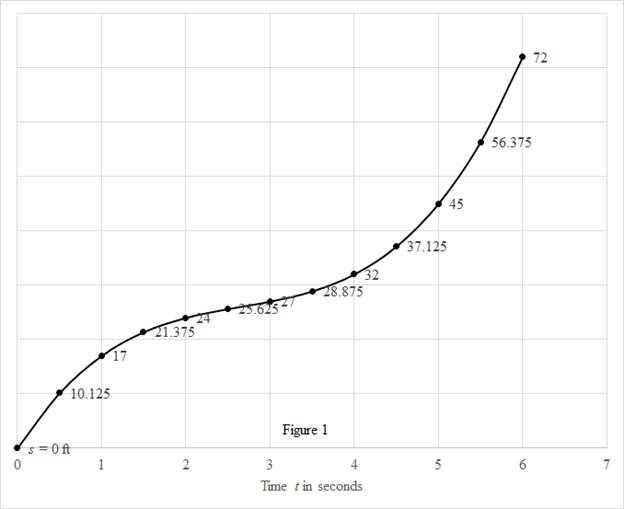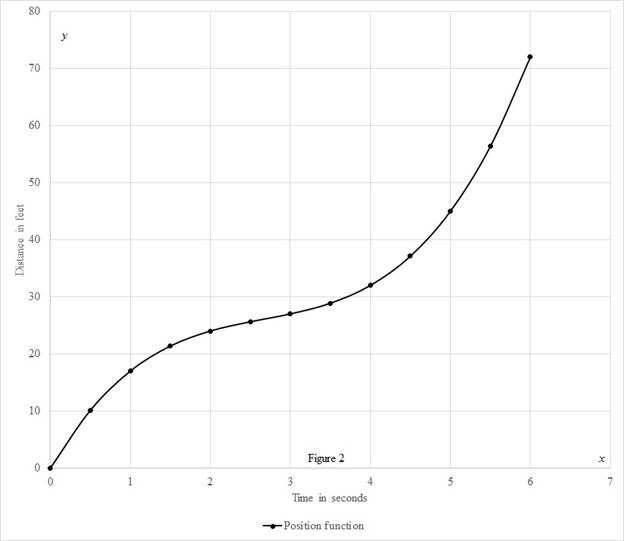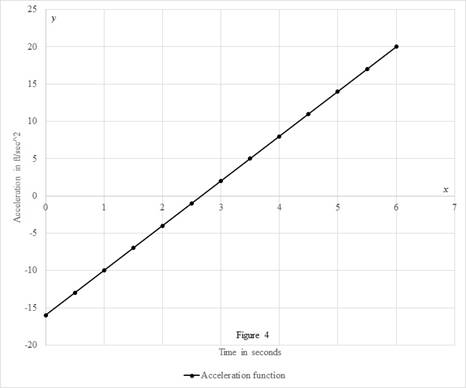# The velocity at time t .### Single Variable Calculus: Concepts...

4th Edition
James Stewart
Publisher: Cengage Learning
ISBN: 9781337687805### Single Variable Calculus: Concepts...

4th Edition
James Stewart
Publisher: Cengage Learning
ISBN: 9781337687805

#### Solutions

Chapter 3.8, Problem 1E

(a)

To determine

## To find: The velocity at time t.

Expert Solution

The velocity at time is 3t216t+24ft/sec_.

### Explanation of Solution

Given:

The given equation is as below.

s=f(t)=t38t2+24t (1)

Calculation:

Calculate the velocity at time t.

Differentiate the equation (1) with respect to time.

v(t)=f'(t)=3t216t+24 (2)

Therefore, the velocity at time t is 3t216t+24ft/sec_.

(b)

To determine

### To find: The velocity after 1 second.

Expert Solution

The velocity after 1 second is v(1)=11ft/sec_.

### Explanation of Solution

Calculate the velocity after 1 second.

Substitute 1 for t in the equation (2).

v(t)=f'(t)=3t216t+24v(1)=f'(1)=3(1)216(1)+24v(1)=11ft/sec

Therefore, the velocity after 1 second is v(1)=11ft/sec_.

(c)

To determine

### To find: The time when particle at rest.

Expert Solution

The particle never is at rest.

### Explanation of Solution

Calculate the time when particle will be at rest.

The velocity will be zero, when the particle is at rest.

Substitute 0 for v(t) in the equation (2).

v(t)=3t216t+240=3t216t+24t=16t±(16)24(3)(24)2(3)=16±326

From the above equation, the value of time t doesn’t exist. Therefore, the particle never is at rest.

(d)

To determine

### To find: The particle moving in the positive direction.

Expert Solution

The velocity of particle always moves in positive direction.

### Explanation of Solution

Calculate the time at which the particle will be moving in the positive direction.

If speed is positive, the particle moves in positive direction whereas the speed is negative, the particle moves in negative direction.

Substitute 0 for t in the equation (2).

v(t)=3t216t+24v(0)=3(0)216(0)+24=24

Therefore, the velocity at t=0 is positive and the velocity never get zero since the value of t will be positive. Hence, the particle will always move in positive direction.

(e)

To determine

### To find: The total distance traveled during the first 6 seconds.

Expert Solution

The total distance travelled during first 6 seconds is f(6)=72ft_.

### Explanation of Solution

Calculate the total distance traveled during first 6 seconds.

Substitute 0 for t in the equation (1).

f(t)=t38t2+24tf(0)=(03)8(0)2+24(0)f(0)=0

Substitute 6 for t in the equation (1).

f(t)=t38t2+24tf(6)=(63)8(6)2+24(6)f(6)=72

Therefore, the total distance travelled during first 6 seconds is f(6)=72ft_.

(f)

To determine

### To find: The diagram to illustrate the motion of the particle.

Expert Solution

The diagram is shown in the figure (1).

### Explanation of Solution

Show the diagram to illustrate the motion of the particle as shown below in figure (1).(g)

To determine

### To find: The acceleration at time t and after 1 second.

Expert Solution

The acceleration at time is f"(t)=6t16_ and after one second is 10ft/s2_.

### Explanation of Solution

Calculate the acceleration at time t.

Differentiate the equation (2) with respect to t.

f'(t)=3t216t+24f"(t)=6t16

Therefore, the acceleration at time is (6t16)ft/s2_.

Calculate the acceleration after 1 second.

Substitute 1 for t in the above equation.

f"(t)=6t16f"(1)=6(1)16=10fts2

Therefore, the acceleration after 1 second is 10ft/s2_

(h)

To determine

Expert Solution

### Explanation of Solution

Calculate the position using the formula.

f(t)=t38t2+24t

Substitute 0 for t in the above equation.

f(t)=t38t2+24tf(0)=(0)38(0)2+24(0)=0

Similarly, calculate the remaining values.

Calculate the value of t and f(t) as shown in the table (1).

 t f(t)=t3−8t2+24t 0 0 0.5 10.125 1 17 1.5 21.375 2 24 2.5 25.625 3 27 3.5 28.875 4 32 4.5 37.125 5 45 5.5 56.375 6 72

Calculate the velocity using the expression.

v(t)=3t216t+24

Substitute 0 for t in the above equation.

v(t)=3t216t+24v(0)=3(0)216(0)+24=24

Similarly, calculate the remaining values.

Calculate the value of t and v(t) as shown in the table (2).

 t v(t)=t3−8t2+24t 0 24 0.5 16.75 1 11 1.5 6.75 2 4 2.5 2.75 3 3 3.5 4.75 4 8 4.5 12.75 5 19 5.5 26.75 6 36

Calculate the acceleration using the formula.

v(t)=6t16

Substitute 0 for t in the above equation.

v(t)=6t16v(0)=6(0)16=16

Similarly, calculate the remaining values.

Calculate the value of t and a(t) as shown in the table (3).

 t a(t)=t3−8t2+24t 0 -16 0.5 Z-13 1 -10 1.5 -7 2 -4 2.5 -1 3 2 3.5 5 4 8 4.5 11 5 14 5.5 17 6 20

Draw the position as a function of time curve as shown in the figure (1).Draw the speed as a function of time curve as shown in the figure (2).Draw the acceleration as a function of time curve as shown in the figure (3).(i)

To determine

### To find: The time when the particle is speeding up and slowing down.

Expert Solution

The time when the particle is speeding up is when time t is greater than 83seconds, and the time when the particle is slowing down is when time is lesser than 83seconds.

### Explanation of Solution

Calculate the time when particle is speeding up and slowing down.

Substitute 0 for f"(t) in the equation f"(t)=6t16.

f"(t)=6t160=6t166t=16t=166t=83sec

Substitute 93sec for t in the equation f"(t)=6t16.

f"(t)=6t16f"(93)=6(93)16=2

Substitute 73sec for t in the equation f"(t)=6t16.

f"(t)=6t16f"(73)=6(73)16=2

Therefore, the acceleration is positive when the value of time is t>83sec and this will be speeding up and the acceleration is negative when the value of time is t<83sec and this will be slowing down.

### Have a homework question?

Subscribe to bartleby learn! Ask subject matter experts 30 homework questions each month. Plus, you’ll have access to millions of step-by-step textbook answers!